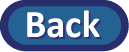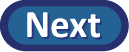## Simulation cont.

To better understand the behavior of this null distribution of sample slopes, we of course need to take a lot more samples.
• Set the Number of Shuffles to 990 (for a total of 1000).
• Press Shuffle Y-values.

(j) Describe the shape, center (mean), and spread (standard deviation) of the histogram for the resulting null distribution of sample slopes. Also, make sure you describe what the standard deviation is measuring (Hint: What is the "variable" in this histogram?).

Now use this distribution to approximate the p-value for our research question.

• For the Count Samples box, use the pull-down menu to indicate whether you want a one-sided (Great Than or Less Than) or a two-sided (Beyond) p-value, and then specify the value of the observed sample slope for this study in the box, and press Count.
(k) Include a screen capture of your output (null distribution and p-value) and provide a one-sentence interpretation of this p-value in the context of the Facebook study. (What is it the probability of and what do we mean by probability?)

(l) What conclusion will you draw from this p-value?

(m) Using the standard deviation you found in (j), how many standard deviations is the observed sample slope (0.2) from the hypothesized slope (0.0)? Show your work.

(n) Also use the standard deviation you found in (j) to approximate a 95% confidence interval for the population slope. (Using the 2SD short-cut.)